# Algebra - One On One 1.1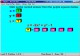Algebra One on One is an educational game for those wanting a fun way to learn and practice Algebra.

 Developer: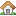Sheppard Software      software by Sheppard Software → Price: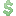14.00  buy → License: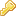Trial File size: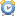0K Language:OS: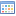Windows Vista (?) Rating:0 /5 (0 votes)

Algebra One on One is an educational game for those wanting a fun way to learn and practice Algebra. This program covers 21 functions which includes maximums, minimums, absolute values, averages, x/y, ax + b, axy + b, ax + by + c, squares, cubes, and so on. It has a practice and a game area.

Algebra One on One is an educational game for those wanting a fun way to learn and practice Algebra. This program covers 21 functions (equations) which includes maximums, minimums, absolute values, averages, x/y, ax + b, axy + b, ax + by + c, squares, cubes, and so on.

You can practice each function individually by choosing Level, Practice Game, and the function you want to practice. The Algebra Game menu under the Level menu lets you choose to one function, two functions, and so on up to 21 functions.

The Ways To Play menu lets you choose from Calculate value, Choose formula, Figure formula and calculate. The calculate value option gives you one x and one y and the equation and you put the x numbers and or the y numbers in the equation and solve.

The Choose formula option gives you several values for x and for y along with answers for each value (z) and it is up to you to determine which equation is being used and click on the right one out of 5 possible answers (equations) listed.

The figure formula gives you several values for x and for y along with answers for all of them except 1 (?) and it is up to you to first determine the function (equation) being used then insert the x and or y values in the equation and solve for "?". If you get stuck trying to figure out what the function (problem) is a hint will be displayed according to the settings you set. Hint settings are wait 5 -60 seconds before displaying hint or No Hint.

You can choose from a ten problem game, a time trial game, or a two player game. High scores are saved and you are given a rank according to your score. The ranks are Novice, Learner, Veteran, Calculator, Math Pro, Math Whiz, Math Genius, and Einstein.

You are given your rank for both the present game and the average score of previous games completed. It offers positive reinforcement in the form of "Correct!!!" displayed in big red letters and playing the windows tada.wav. If you choose a wrong answer it will say "oh" and put a red X over the wrong answer then you choose another answer if that one is wrong also it will do the same thing until you click on the correct answer. The numbers are displayed in colorful boxes. There is also a pink box in the upper left corner that click to go on to the next problem.

The calculate option combined with the practice game is a definite plus in this game enabling students practice solving the problems in an specific area they are having trouble with. Also this may be meant for middle school students but it is a good program to prepare your little ones for algebra and help them also practice their basic math skills.

I play the game with my 10 year old breaking down each level of the problem solving he has to do the calculations but at the same times is able to see what I am doing. In other words if x = 8 and the problem is x squared plus 2 then I ask him what 8 times 8 is so he sees that x squared means to times the number by itself. This is a truly great program for people of all ages.

Here are some key features of "Algebra One On One":

• Makes algebra fun
• Over six different play options insure you'll never be bored
• Allows exciting head - to - head competition between two players
• Builds your skills step-by-step - has over 21 levels
• Has millions and millions of problems - you'll never get the same problem twice

###### Limitations
• 30 day trial
• Nag screen

tags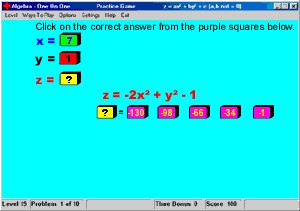# Download Algebra - One On One 1.1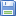Download Algebra - One On One 1.1

Purchase: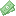Buy Algebra - One On One 1.1

###### Similar softwareAlgebra - One On One 1.1
Sheppard Software

Algebra One on One is an educational game for those wanting a fun way to learn and practice Algebra.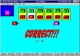Math Function Mania 3.0
Sheppard Software

Math Function Mania is a multimedia game that help you to learn functions, algebra and how to solve problems.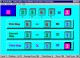Brain Builder - Math Edition 3.0
Sheppard Software

Brain Builder - Math Edition is a game that includes over 500 million problems presented in a fun multimedia format.Math Basics 0.1
KIDware

Math Basicshelp will help you learn the four basic arithmetic operations of addition, subtraction, multiplication, and division for whole numbers, fractions, and decimals.Chemistry Games, Labs, & Demos 1.0
Multimedia Science

Chemistry Games, Labs, & Demos is a useful set of tutorials, labs, demonstrations, and games on basic chemistry topics.WhizKid Math Flashcards 1.0
Skye Publications

WhizKid Math Flashcards combines the look and feel of traditional math flashcards with the flexibility and ease of a piece of software.Master Math Word Problems 1.2
Fast Rabbit Software, LLC

Solving word problems is an area where elementary students overwhelmingly display difficulties.WordLab 1.12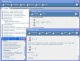EMMentor Algebra short 3.0Ultimate Math Solver 6.0.2.3